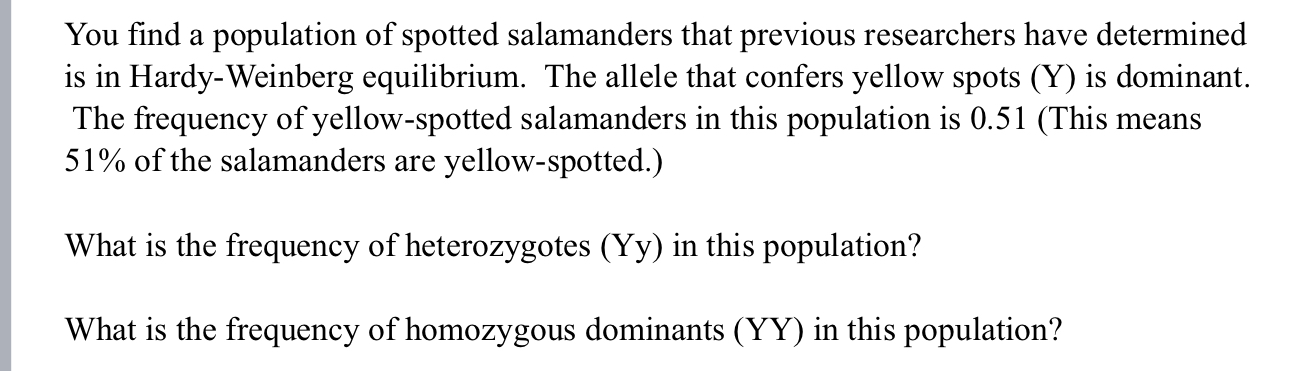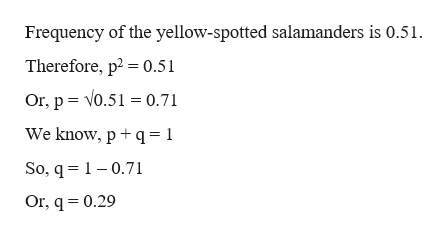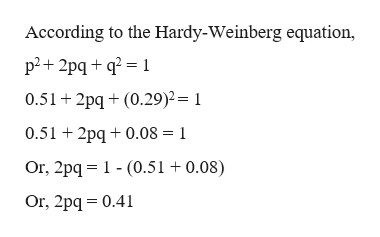# You find a population of spotted salamanders that previous researchers have determinedis in Hardy-Weinberg equilibrium. The allele that confers yellow spots (Y) is dominantThe frequency of yellow-spotted salamanders in this population is 0.51 (This means51% of the salamanders areyellow-spotted.)What is the frequency of heterozygotes (Yy) in this population?What is the frequency of homozygous dominants (YY) in this population?

Questionhelp_outlineImage TranscriptioncloseYou find a population of spotted salamanders that previous researchers have determined is in Hardy-Weinberg equilibrium. The allele that confers yellow spots (Y) is dominant The frequency of yellow-spotted salamanders in this population is 0.51 (This means 51% of the salamanders are yellow-spotted.) What is the frequency of heterozygotes (Yy) in this population? What is the frequency of homozygous dominants (YY) in this population? fullscreen
check_circle

Step 1

The Hardy-Weinberg equation is a mathematical equation that can be used to calculate the genetic variation of a population at equilibrium. The Hardy-Weinberg equation is expressed as:

p2 + 2pq + q2 = 1

where, p is the frequency of the dominant allele and q is the frequency of the recessive allele in the population. In the equation, p2 represents the frequency of the homozygous dominant genotype, q2 represents the frequency of the homozygous recessive genotype, and 2pq represents the frequency of the heterozygous genotype. In addition, the sum of the allele frequencies for all the alleles at the locus must be 1. So,

p + q =1

Step 2

The homozygous dominant allele and the homozygous recessive allele are represented as p and q respectively.help_outlineImage TranscriptioncloseFrequency of the yellow-spotted salamanders is 0.51 Therefore, p2 0.51 Or, p V0.5 0.71 We know, p q 1 So, q 10.71 Or, q 0.29 fullscreen
Step 3

The frequency of the dominant allele (p) is 0.71 and that of the recessive allele (q) is 0.29. The frequency ...help_outlineImage TranscriptioncloseAccording to the Hardy-Weinberg equation p2 2pqq2 1 0.51 2pq(0.29)2= 1 0.51 2pq0.08 1 Or, 2pq (0.51 + 0.08) Or, 2pq 0.41 fullscreen

### Want to see the full answer?

See Solution

#### Want to see this answer and more?

Solutions are written by subject experts who are available 24/7. Questions are typically answered within 1 hour.*

See Solution
*Response times may vary by subject and question.
Tagged in

### Biology